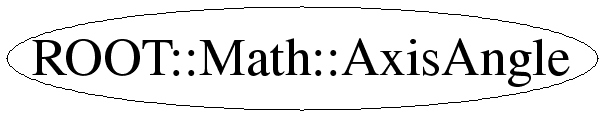# class ROOT::Math::AxisAngle

```
AxisAngle class describing rotation represented with direction axis (3D Vector) and an
angle of rotation around that axis.

@ingroup GenVector

```

## Function Members (Methods)

public:
 ~AxisAngle() ROOT::Math::AxisAngle::Scalar Angle() const ROOT::Math::AxisAngle::AxisVector Axis() const ROOT::Math::AxisAngle AxisAngle() ROOT::Math::AxisAngle AxisAngle(const ROOT::Math::AxisAngle&) ROOT::Math::AxisAngle AxisAngle(const ROOT::Math::DisplacementVector3D,ROOT::Math::DefaultCoordinateSystemTag>& v, ROOT::Math::AxisAngle::Scalar angle) ROOT::Math::AxisAngle AxisAngle(double* begin, double* end) void GetComponents(double* begin) const ROOT::Math::AxisAngle Inverse() const void Invert() bool operator!=(const ROOT::Math::AxisAngle& rhs) const ROOT::Math::AxisAngle::XYZVector operator()(const ROOT::Math::AxisAngle::XYZVector& v) const ROOT::Math::AxisAngle operator*(const ROOT::Math::Rotation3D& r) const ROOT::Math::AxisAngle operator*(const ROOT::Math::AxisAngle& a) const ROOT::Math::AxisAngle operator*(const ROOT::Math::EulerAngles& e) const ROOT::Math::AxisAngle operator*(const ROOT::Math::Quaternion& q) const ROOT::Math::AxisAngle operator*(const ROOT::Math::RotationZYX& r) const ROOT::Math::AxisAngle operator*(const ROOT::Math::RotationX& rx) const ROOT::Math::AxisAngle operator*(const ROOT::Math::RotationY& ry) const ROOT::Math::AxisAngle operator*(const ROOT::Math::RotationZ& rz) const ROOT::Math::PositionVector3D,ROOT::Math::DefaultCoordinateSystemTag> operator*(const ROOT::Math::PositionVector3D,ROOT::Math::DefaultCoordinateSystemTag>& v) const ROOT::Math::DisplacementVector3D,ROOT::Math::DefaultCoordinateSystemTag> operator*(const ROOT::Math::DisplacementVector3D,ROOT::Math::DefaultCoordinateSystemTag>& v) const ROOT::Math::LorentzVector > operator*(const ROOT::Math::LorentzVector >& v) const ROOT::Math::AxisAngle& operator=(ROOT::Math::Rotation3D const& r) ROOT::Math::AxisAngle& operator=(ROOT::Math::RotationX const& r) ROOT::Math::AxisAngle& operator=(ROOT::Math::RotationY const& r) ROOT::Math::AxisAngle& operator=(ROOT::Math::RotationZ const& r) ROOT::Math::AxisAngle& operator=(ROOT::Math::Quaternion const& r) ROOT::Math::AxisAngle& operator=(const ROOT::Math::AxisAngle&) bool operator==(const ROOT::Math::AxisAngle& rhs) const void Rectify() void SetComponents(double* begin, double* end)
private:
 static double Pi() void RectifyAngle()

## Data Members

private:
 ROOT::Math::AxisAngle::Scalar fAngle rotation angle ROOT::Math::AxisAngle::AxisVector fAxis rotation axis (3D vector)

## Class Charts## Function documentation

AxisAngle(const ROOT::Math::AxisAngle& )
```Default constructor (axis is z and angle is zero)

```
`{ }`
AxisAngle(const AnyVector & v, Scalar angle)
```Construct from a non-zero vector (x,y,z) and an angle.
Precondition:  the Vector needs to implement x(), y(), z(), and unit()

```
`{ }`
AxisAngle(IT begin, IT end)
```Construct given a pair of pointers or iterators defining the
beginning and end of an array of four Scalars, to be treated as
the x, y, and z components of a unit axis vector, and the angle
of rotation.
Precondition:  The first three components are assumed to represent
the rotation axis vector and the 4-th the rotation angle.
The angle is assumed to be in the range (-pi,pi].
The axis vector is automatically normalized to be a unit vector

```
`{ SetComponents(begin,end); }`
void Rectify()
``` The compiler-generated copy ctor, copy assignment, and dtor are OK.

Re-adjust components to eliminate small deviations from the axis
being a unit vector and angles out of the canonical range (-pi,pi]

```
explicit AxisAngle(const ROOT::Math::AxisAngle& )
``` ======== Construction From other Rotation Forms ==================

Construct from another supported rotation type (see gv_detail::convert )

```
`{gv_detail::convert(r,*this);}`
AxisAngle & operator=( OtherRotation const & r )
```Assign from another supported rotation type (see gv_detail::convert )

```
void SetComponents(double* begin, double* end)
``` ======== Components ==============

Set the axis and then the angle given a pair of pointers or iterators
defining the beginning and end of an array of four Scalars.
Precondition:  The first three components are assumed to represent
the rotation axis vector and the 4-th the rotation angle.
The angle is assumed to be in the range (-pi,pi].
The axis vector is automatically normalized to be a unit vector

```
void GetComponents(IT begin, IT end)
```Get the axis and then the angle into data specified by an iterator begin
and another to the end of the desired data (4 past start).

```
Scalar Angle()
```access to rotation angle

```
`{ return fAngle; }`
XYZVector operator()(const ROOT::Math::AxisAngle::XYZVector& v) const
AVector operator*(const AVector & v)
```Overload operator * for rotation on a vector

```
void Invert()
```Invert an AxisAngle rotation in place

```
`{ fAngle = -fAngle; }`

```Return inverse of an AxisAngle rotation

```
`{ AxisAngle result(*this); result.Invert(); return result; }`
return ! operator==(const ROOT::Math::AxisAngle& rhs) const
void RectifyAngle()
double Pi()
`{ return 3.14159265358979323; }`
AxisAngle operator*(RotationX const & r1, AxisAngle const & r2)
```Multiplication of an axial rotation by an AxisAngle

```
AxisAngle operator*(RotationY const & r1, AxisAngle const & r2)
AxisAngle operator*(RotationZ const & r1, AxisAngle const & r2)

Last update: root/mathcore:\$Id: AxisAngle.h 21503 2007-12-19 17:34:54Z moneta \$
Copyright (c) 2005 , LCG ROOT MathLib Team *

This page has been automatically generated. If you have any comments or suggestions about the page layout send a mail to ROOT support, or contact the developers with any questions or problems regarding ROOT.